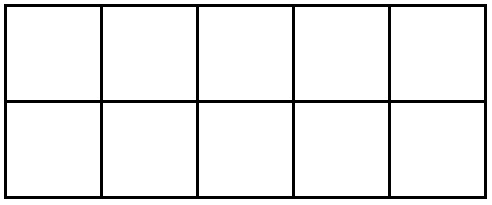Ten-frames are a simple graphic tool that allow children to “see” number values.  They are used in Pre-K through 2nd grade to represent values, build an understanding of ten, relate ten to place value in numbers greater than ten, help students to use and understand “make-a-ten” strategies in adding and subtracting, and eventually to represent groups of ten ones in multi-digit addition and subtraction.  (That sure is powerful for a simple tool!)

Adding a second ten-frame to create a double ten-frame can also be useful for modeling teen numbers through twenty. Children can then add more ten-frames to model numbers 20-100. Ten-frames are fantastic representational models to help students understand and work with our base-ten number system.

Ten frames can be found in various forms, but they basically look like this…This is how a student might represent the values of 4 and 7 using counters on a ten frame…Visit this interactive ten-frame for four different activities involving the ten-frame.  Even though it is not stated, the final activity uses a “make-a-ten” strategy for adding.  Students will break apart an addend to fill the first ten-frame, then count 10 and how many more to find their sum.

Also, you may wish to visit this Animated Math Model from Houghton Mifflin.